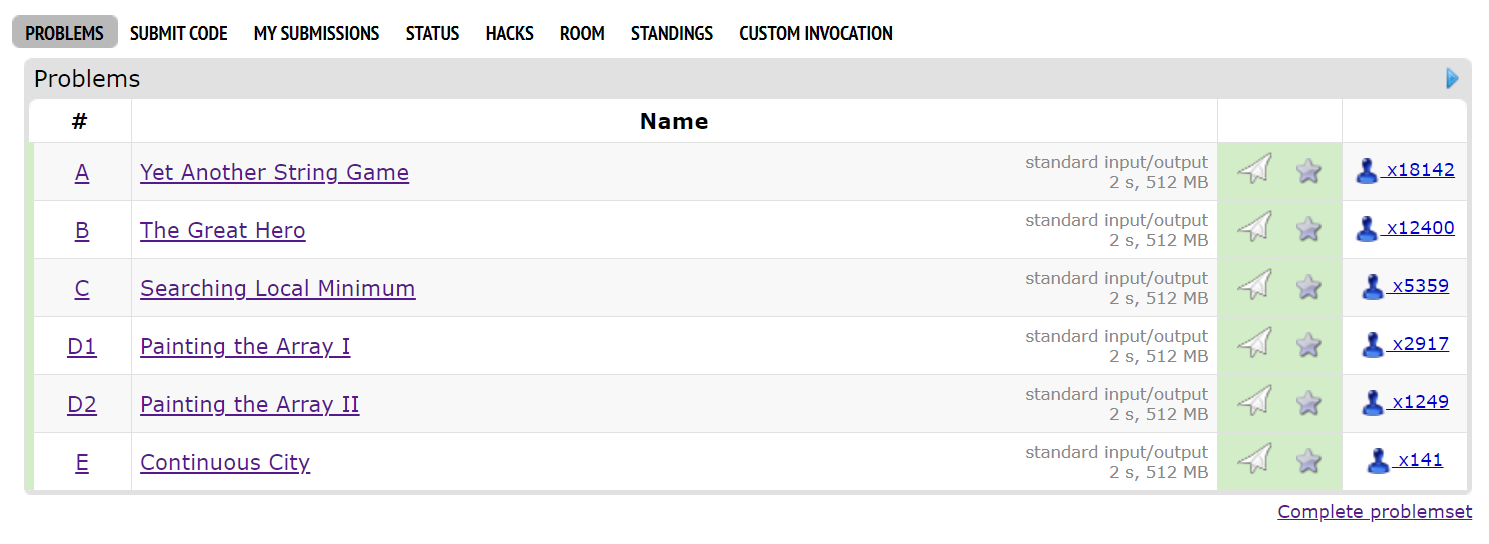## Codeforces Round #700 (Div. 2) 题解Round 700 Div.2给人的感觉是手速场，基本上没有什么很需要思考的点。

## [DP][字符串匹配][子序列] codeforces 1150 D Three Religions

vp了这一场，这个D题难到出现断层。。。C过了1k人的时候D没人过，太狠了

vp的时候毫无头绪，正解里的预处理表倒是想到了也写了但是没用上。确实没想到会是dp emmmm 。既然dp那一定有个数值型的对象吧，他这里就很妙的把问题转化为满足这三个subsequence共存的序列至少要多长了。。。当然给定的word of universe是确定的，这里其实可以认为后面还有无限长的字符串。。。但是长度大于n的时候我们认为没用了。。就输出NO就可以。不得不说确实是很精妙，legendary出的题确实厉害👍

$dp\left(n_{1}, n_{2}, n_{3}\right)=\min \left\{\begin{array}{ll}{Nst\left(dp\left(n_{1}-1, n_{2}, n_{3}\right)+1, \text{description}_{1}\left[n_{1}\right]\right)} & {\text { if } n_{1} \geq 1} \\ {Nst\left(dp\left(n_{1}, n_{2}-1, n_{3}\right)+1, \text{description}_{2}\left[n_{2}\right]\right)} & {\text { if } n_{2} \geq 1} \\ {Nst\left(dp\left(n_{1}, n_{2}, n_{3}-1\right)+1, \text { description }_{3}\left[n_{3}\right]\right)} & {\text { if } n_{3} \geq 1}\end{array}\right.$

## [数论][二次剩余应用][组合数][等比数列] ZOJ 3774 Power of Fibonacci继续阅读“[数论][二次剩余应用][组合数][等比数列] ZOJ 3774 Power of Fibonacci”

## [CF][二分check][贪心][×] codeforces 1132D Stressful Training## [线段树+][LCA][dfs序][二分查找][×] codeforces 1062E

http://codeforces.com/contest/1062/problem/E